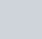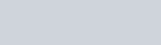# Hogwarts Legacy Door Puzzles Explained SIMPLEHogwarts Legacy Door Puzzles Explained, this puzzles have specific rules, and are a common type of mathematical puzzle, that require mathematics to find a solution that satisfies the given conditions. After these conditions are set, the rules of the puzzle determine all subsequent changes and moves.

We know that there are people who have a hard time finishing the Hogwarts Legacy game. If you are one of those who find it difficult to finish the game, let’s take you to our Hogwarts Legacy guide.

## Hogwarts Legacy Door Puzzles Explained Simple Way

Welcome to our Hogwarts Legacy Mathematics Door Puzzles guide. Mathematic Door Puzzles in Hogwarts Legacy have specific rules, and are a common type of mathematical puzzle, that require mathematics to find a solution that satisfies the given conditions. After these conditions are set, the rules of the puzzle determine all subsequent changes and moves.

### How to Solve Door Puzzles in Hogwarts Legacy

To solve the mathematical puzzle, we first need to find the solution to a simple mathematical equation that we can observe in each of the pyramids in the center of the puzzle. Each of the vertices of the pyramid represents a number, and the sum of each must result in the number of the center.

### Numbers and Figures Chart

In order to interpret what number each carved figure represents, you can simply verify it in the following image.

### How to Place the Missing Figures

To place the correct figure we must use your magic wand to activate and move the two mechanisms that are on each of the sides of the great puzzle until you find the correct figure.

Each of these mechanisms corresponds to each equation. The pyramid on the left acts on the first equation, while the mechanism on the right acts on the second of the equations.

### Mathematic Door Puzzles

These types of puzzles are a unique and exciting feature, that will test your math skills

#### First equation:

The upper pyramid contains the first of the equations. It is the simplest, since we only need to solve what value represents the sign3 + 0 + = 5
This means: 3 + 0 + 2 = 5
= 2
The missing figure that represent the number 2 isIn this case, the missing number is 2, represented by The Graphorn figure.

#### Second Equation:

The inverted pyramid contains the second of the equations and is somewhat more complex, since in addition to the numbers in the vertices, we also observe a figure of a unicorn. Each figure carved in the frame that surrounds the entire wooden door is equivalent to a number.

4 +  + = 12
== 7
The missing figure that represent the number 7 is